# 19. For each of the three cases, plot the particular solution as a function of time...

19. For each of the three cases, plot the particular solution as a function of time in MATLAB. You should get a similar graphs to the

Hey dear I think there is some technical issue in uploading the question . Only a certain part of the question is visible. So I humbly request you to upload the full question so that you can be helped with the answer. Thank you.

No cases are given and the question is also not full.

#### Earn Coin

Coins can be redeemed for fabulous gifts.

Similar Homework Help Questions
• ### Useaplottingroutinetoexaminethebasemotionproblem(seeFigure2.13)byplotting the particular solution (for an undamped system) for the three cases k = 1500 N>m,...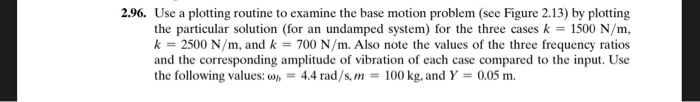Useaplottingroutinetoexaminethebasemotionproblem(seeFigure2.13)byplotting the particular solution (for an undamped system) for the three cases k = 1500 N>m, k = 2500 N>m, and k = 700 N>m. Also note the values of the three frequency ratios and the corresponding amplitude of vibration of each case compared to the input. Use the following values: ωb = 4.4 rad>s, m = 100 kg, and Y = 0.05 m. PLEASE PROVIDE THE MATLAB CODE 2.96. Use a plotting routine to examine the base motion problem...

• ### Make three separate plots, each plot containing two graphs at different temperatures. Plot each set for...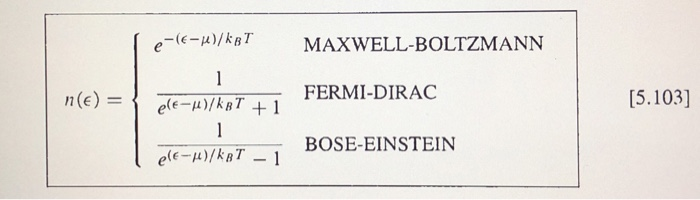Make three separate plots, each plot containing two graphs at different temperatures. Plot each set for equations 5.103 as a function of ? − ? , for two separate temperatures close to absolute zero, say 0.1 K and 0.001 K to show the general trend of these objects. Label everything! NOTE: Be super careful with the Fermi-Dirac distribution graph. Make sure you explain what you are doing and why. e-((-μ)/kaT MAXWELL-BOLTZMANN FERMI-DIRAC BOSE-EINSTEIN [5.103] ele-μ)/hT-1

• ### 7 11. If a particular network is described by transfer function H(s), use MATLAB to plot the magnitude and phase Bode plot for H(s) equal to (a) 300 (s2 7s +7 s(5s +8s(2s + 4) 7 11. If a particu...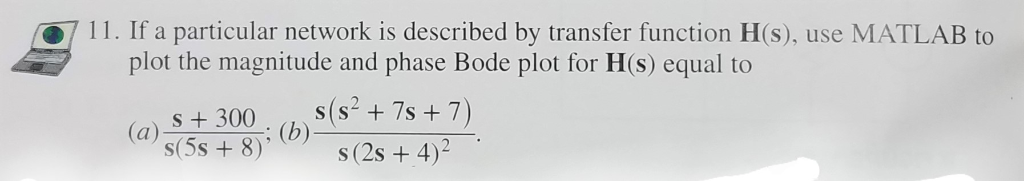7 11. If a particular network is described by transfer function H(s), use MATLAB to plot the magnitude and phase Bode plot for H(s) equal to (a) 300 (s2 7s +7 s(5s +8s(2s + 4) 7 11. If a particular network is described by transfer function H(s), use MATLAB to plot the magnitude and phase Bode plot for H(s) equal to (a) 300 (s2 7s +7 s(5s +8s(2s + 4)

• ### 2. Plot the concentrations listed below as a function of time. Then, plot any other graphs...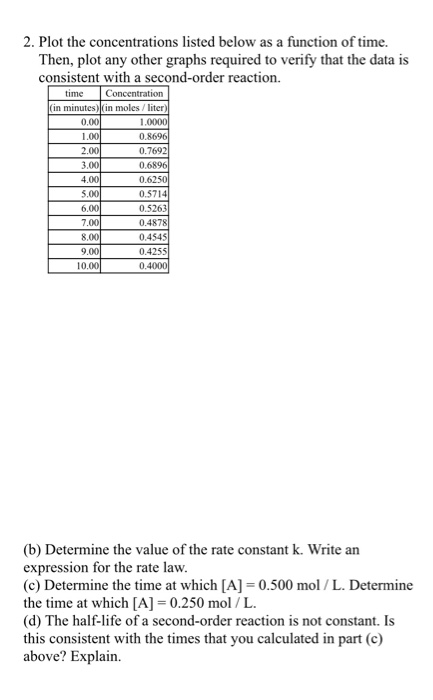2. Plot the concentrations listed below as a function of time. Then, plot any other graphs required to verify that the data is consistent with a second-order reaction. time Concentration (in minutes)(in moles/liter) 0.00 1.0000 1.00 0.8696 2.00 0.7692 3.00 0.6896 4.00 0.6250 5.00 0.5714 6.00 0.5263 7.00 0.4878 8.00 0.4545 9.00 0.4255 10.00 0.40001 (b) Determine the value of the rate constant k. Write an expression for the rate law. (c) Determine the time at which [A] =0.500 mol/L....

• ### Plot the concentrations listed below as a function of time. Then, plot any other graphs required...

Plot the concentrations listed below as a function of time. Then, plot any other graphs required to verify that the data is consistent with a second-order reaction. time Concentration (in minutes) (in moles / liter) 0.00 1.0000 1.00 0.8696 2.00 0.7692 3.00 0.6896 4.00 0.6250 5.00 0.5714 6.00 0.5263 7.00 0.4878 8.00 0.4545 9.00 0.4255 10.00 0.4000 (b) Determine the value of the rate constant k. Write an expression for the rate law. (c) Determine the time at which [A]...

• ### A classic second order system has transfer function the undamped natural frequency to be 10 rad/s throughout this exercise. Note, for the following MATLAB simulations you need to use format long defi...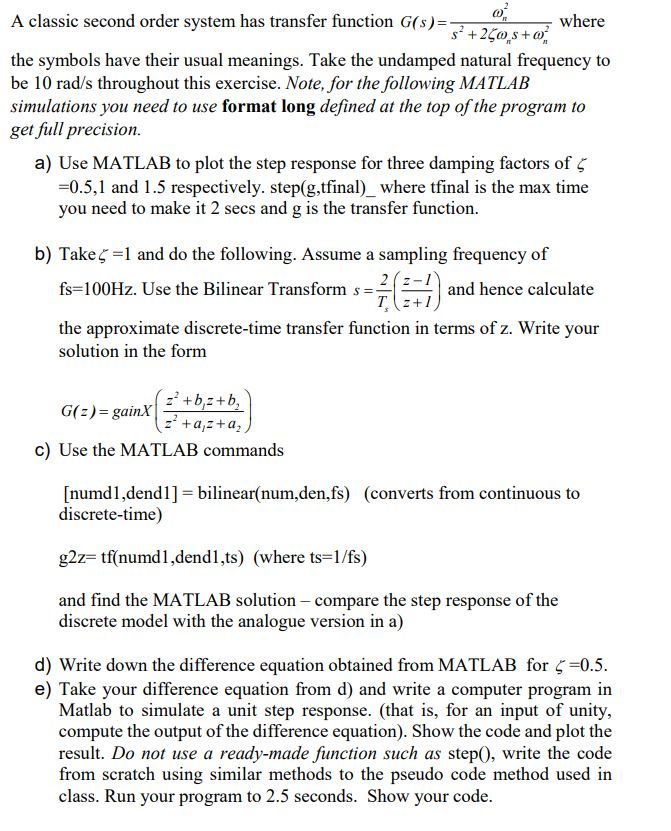A classic second order system has transfer function the undamped natural frequency to be 10 rad/s throughout this exercise. Note, for the following MATLAB simulations you need to use format long defined at the top of the program to get full precision. a) Use MATLAB to plot the step response for three damping factors of ζ =0.5,1 and 1.5 respectively. step(g,tfinal)_ where tfinal is the max time you need to make it 2 secs and g is the b) Takeζ...

• ### In MATLAB please Consider the nonlinear function: y = f(x) = x3 cos x a. Plot...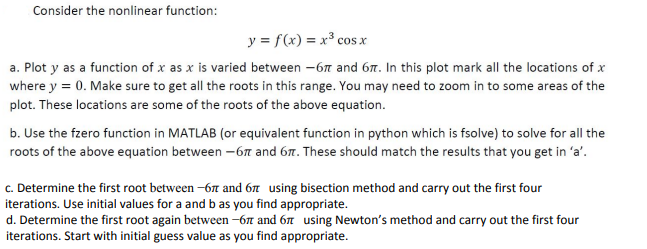In MATLAB please Consider the nonlinear function: y = f(x) = x3 cos x a. Plot y as a function of x as x is varied between -67 and 67. In this plot mark all the locations of x where y = 0. Make sure to get all the roots in this range. You may need to zoom in to some areas of the plot. These locations are some of the roots of the above equation. b. Use the fzero...

• ### please write code in matlab thankyou (10 points) Plot the function f(x) = (3cos-sinx)e-02x for-4 appropriate...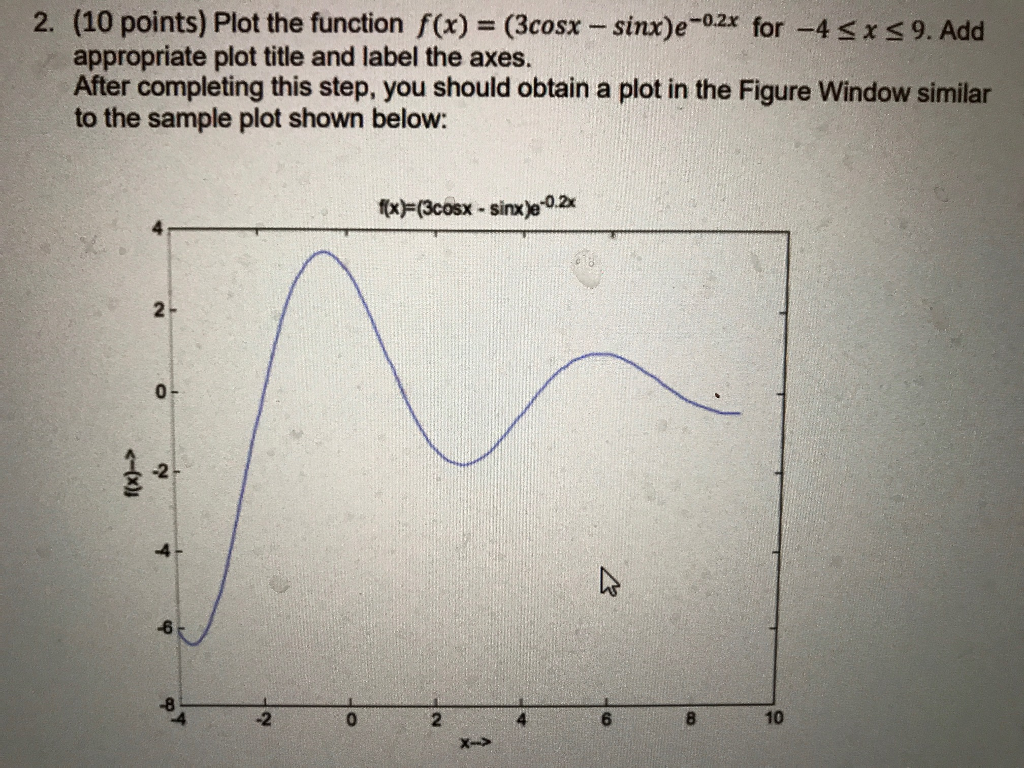please write code in matlab thankyou (10 points) Plot the function f(x) = (3cos-sinx)e-02x for-4 appropriate plot title and label the axes. After completing this step, you should obtain a plot in the Figure Window similar to the sample plot shown below: 2. 9. Add 0.2x 2- 0- -2 -8 -2 2 6 10

• ### 1. (a) Plot the concentrations listed below as a function of time. Then, plot any other...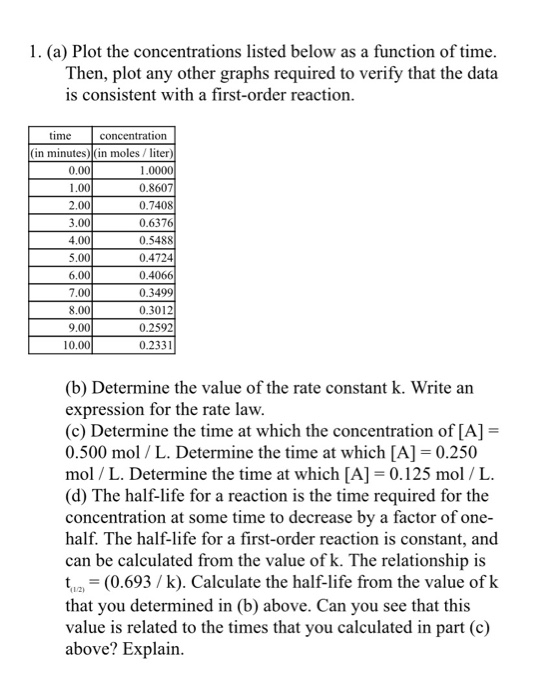1. (a) Plot the concentrations listed below as a function of time. Then, plot any other graphs required to verify that the data is consistent with a first-order reaction. time concentration (in minutes) (in moles / liter) 0.00 1.0000 1.00 0.8607 2.00 0.7408 3.00 0.6376 4.00 0.5488 5.00 0.4724 6.00 0.4066 7.00 0.3499 8.00 0.3012 9.00 0.2592 10.00 0.2331 (b) Determine the value of the rate constant k. Write an expression for the rate law. (c) Determine the time at...

• ### (a) Plot the concentrations listed below as a function of time. Then, plot any other graphs...

(a) Plot the concentrations listed below as a function of time. Then, plot any other graphs required to verify that the data is consistent with a first-order reaction. time concentration (in minutes) (in moles / liter) 0.00 1.0000 1.00 0.8607 2.00 0.7408 3.00 0.6376 4.00 0.5488 5.00 0.4724 6.00 0.4066 7.00 0.3499 8.00 0.3012 9.00 0.2592 10.00 0.2331 (b) Determine the value of the rate constant k. Write an expression for the rate law. (c) Determine the time at which...